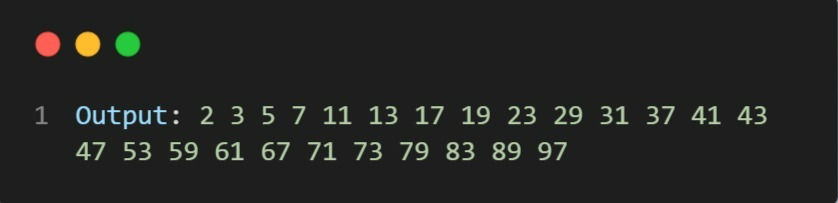top of page

# Program to print prime numbers from 1 to N.

Given a number N, the task is to print the prime numbers from 1 to N.

Examples:

```Input: N = 10
Output: 2, 3, 5, 7```

Algorithm ( Steps):

• First, take the number N as input.

• Then use a for loop to iterate the numbers from 1 to N

• Then check for each number to be a prime number. If it is a prime number, print it

Approach: Now, according to the formal definition, a number ‘n’ is prime if it is not divisible by any number other than 1 and n. In other words, a number is prime if it is not divisible by any number from 2 to n-1

Implementation:

1. Create a function called isPrime that takes a parameter n

def isPrime(n):

2. Since 0 and 1 is not prime return false

if(n == 1 or n == 0): return False

3. Run a loop from 2 to n-1, if the number is divisible by i, then n is not a prime number.

for i in range(2, n): if(n % i == 0): return False

4. otherwise, n is prime number.

`            return True		`

The function is created.....

5. Take an N numbers.

N = 100

6. check for every number from 1 to N

`     for i in range(1, N+1):		`

7. check if the current number is prime and print it.

```	    if(isPrime(i)):
print(i, end=" ")```

Source Code:

Code:Output: